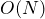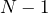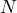# B. Lees & L. Taggi: Exponential decay of correlations in spin and loop O(N) models

Abstract: The spinmodel is an important statistical mechanics model whose spins are vectors taking value in adimensional sphere. The rigorous understanding of such a model is still quite unsatisfactory, especially in thecase. This talk will introduce a random loop representation the model, which is an intriguing mathematical object on its own and includes other important models, such as the dimer model, the double dimer model, the loopmodel. This representation will be used to prove new results about exponential decay of correlations in the presence of an external magnetic field which are valid for any value of, extending previous results

In the first talk we will introduce the spinmodel as well as a general loop model. For a particular choice of parameters this loop model can be connected to the spinmodel (as well as the loopmodel, the dimer and double dimer model, spatial random permutations…). By considering lengths of walks in this loop model we can prove exponential decay of correlations in the spinmodel.

In the second talk we will see how the loop model and spinmodel with external field are connected, and then see the main steps of the proof. In particular we will present a so-called “colour switching” lemma, that allows us to write spin-spin correlations as the expectation of a simple random variable in the loop model. The proof relies only on properties of the loop model and so can be applied quite generally to (for example) inhomogeneous couplings and/or external fields, as well as other related models such as the loopmodel.

## Video